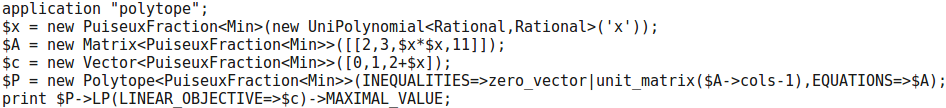# Problem 20

Fix the Puiseux series field $$R = \mathbb{R}\{\!\{ \epsilon \}\!\}$$. Pick a matrix $$A \in \mathbb{R}^{4 \times 8}$$, column vector $$b \in R^4$$ and row vector $$c \in R^8$$, with entries that look random, with powers of $$\epsilon$$. Solve the linear programming problem

Maximize $$c \cdot x$$ subject to $$A x = b$$ and $$x \geq 0$$

## Solution

In Polymake:

application "polytope";
$x = new PuiseuxFraction<Min>(new UniPolynomial<Rational,Rational>('x'));$A = new Matrix<PuiseuxFraction<Min>>([[2,3,$x*$x,11]]);
$c = new Vector<PuiseuxFraction<Min>>([0,1,2+$x]);
$P = new Polytope<PuiseuxFraction<Min>>(INEQUALITIES=>zero_vector|unit_matrix($A->cols-1),EQUATIONS=>$A); print$P->LP(LINEAR_OBJECTIVE=>\$c)->MAXIMAL_VALUE;